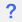super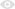14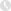Feb 21, 2018

class Base(object):
def __init__(self):
print('-----Base----')

class A(Base):
def __init__(self):
print('------A-----')
super().__init__()

class B(Base):
def __init__(self):
print('-----B----')
super().__init__()

class C(A,B):
def __init__(self):
print('----C----')
super().__init__()

c = C()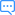回答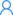whit回答

----C----

------A-----

-----Base----

def super(cls,self):
mro = self.__class__.mro()
return mro[mro.index(cls) + 1](0)